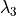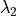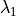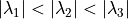# Segment Blood Vessels#

## Synopsis#

The example takes an image (say MRA image), computes the vesselness measure of the image using the HessianRecursiveGaussianImageFilter and the Hessian3DToVesselnessMeasureImageFilter. The goal is to detect bright, tubular structures in the image.

Note that since the algorithm is based on the Hessian, it will also identify black tubular structures.

An important parameter is the Sigma that determines the amount of smoothing applied during Hessian estimation. A larger Sigma will decrease the identification of noise or small structures as vessels. In this example, the Sigma is large enough only vessels comprising the Circle of Willis and other large vessels are segmented.

## Code#

### Python#

#!/usr/bin/env python

import argparse

import itk
from distutils.version import StrictVersion as VS

if VS(itk.Version.GetITKVersion()) < VS("5.0.0"):
print("ITK 5.0.0 or newer is required.")
sys.exit(1)

parser = argparse.ArgumentParser(description="Segment blood vessels.")
args = parser.parse_args()

input_image = itk.imread(args.input_image, itk.ctype("float"))

hessian_image = itk.hessian_recursive_gaussian_image_filter(
input_image, sigma=args.sigma
)

vesselness_filter = itk.Hessian3DToVesselnessMeasureImageFilter[
itk.ctype("float")
].New()
vesselness_filter.SetInput(hessian_image)
vesselness_filter.SetAlpha1(args.alpha1)
vesselness_filter.SetAlpha2(args.alpha2)

itk.imwrite(vesselness_filter, args.output_image)


### C++#

#include "itkHessian3DToVesselnessMeasureImageFilter.h"
#include "itkHessianRecursiveGaussianImageFilter.h"
#include "itkImageFileWriter.h"

int
main(int argc, char * argv[])
{
if (argc < 3)
{
std::cerr << "Usage: <InputImage> <OutputImage> [Sigma] [Alpha1] [Alpha2]" << std::endl;
return EXIT_FAILURE;
}
const char * inputImage = argv;
const char * outputImage = argv;
double       sigma = 1.0;
if (argc > 3)
{
sigma = std::atof(argv);
}
double alpha1 = 0.5;
if (argc > 4)
{
alpha1 = std::atof(argv);
}
double alpha2 = 2.0;
if (argc > 5)
{
alpha2 = std::atof(argv);
}

constexpr unsigned int Dimension = 3;
using PixelType = float;
using ImageType = itk::Image<PixelType, Dimension>;

const auto input = itk::ReadImage<ImageType>(inputImage);

using HessianFilterType = itk::HessianRecursiveGaussianImageFilter<ImageType>;
auto hessianFilter = HessianFilterType::New();
hessianFilter->SetInput(input);
hessianFilter->SetSigma(sigma);

using VesselnessMeasureFilterType = itk::Hessian3DToVesselnessMeasureImageFilter<PixelType>;
auto vesselnessFilter = VesselnessMeasureFilterType::New();
vesselnessFilter->SetInput(hessianFilter->GetOutput());
vesselnessFilter->SetAlpha1(alpha1);
vesselnessFilter->SetAlpha2(alpha2);

try
{
itk::WriteImage(vesselnessFilter->GetOutput(), outputImage);
}
catch (const itk::ExceptionObject & error)
{
std::cerr << "Error: " << error << std::endl;
return EXIT_FAILURE;
}

return EXIT_SUCCESS;
}


## Classes demonstrated#

template<typename TPixel>
class Hessian3DToVesselnessMeasureImageFilter : public itk::ImageToImageFilter<Image<SymmetricSecondRankTensor<double, 3>, 3>, Image<TPixel, 3>>

Line filter to provide a vesselness measure for tubular objects from the hessian matrix.

The filter takes as input an image of hessian pixels (SymmetricSecondRankTensor pixels) and preserves pixels that have eigen valuesclose to 0 andandas large negative values (for bright tubular structures).• Bright tubular structures will have lowand large negative values ofand.

• Conversely dark tubular structures will have a low value ofand large positive values ofand.

• Bright plate like structures have low values ofandand large negative values of• Dark plate like structures have low values ofandand large positive values of• Bright spherical (blob) like structures have all three eigen values as large negative numbers

• Dark spherical (blob) like structures have all three eigen values as large positive numbers

This filter is used to discriminate the Bright tubular structures.

Notes:

The filter takes into account that the eigen values play a crucial role in discriminating shape and orientation of structures.

http://www.image.med.osaka-u.ac.jp/member/yoshi/paper/linefilter.pdf

References:

”3D Multi-scale line filter for segmentation and visualization of

curvilinear structures in medical images”, Yoshinobu Sato, Shin Nakajima, Hideki Atsumi, Thomas Koller, Guido Gerig, Shigeyuki Yoshida, Ron Kikinis.

See

HessianRecursiveGaussianImageFilter

See

SymmetricEigenAnalysisImageFilter

See

SymmetricSecondRankTensor

See itk::Hessian3DToVesselnessMeasureImageFilter for additional documentation.
template<typename TInputImage, typename TOutputImage = Image<SymmetricSecondRankTensor<typename NumericTraits<typename TInputImage::PixelType>::RealType, TInputImage::ImageDimension>, TInputImage::ImageDimension>>
class HessianRecursiveGaussianImageFilter : public itk::ImageToImageFilter<TInputImage, TOutputImage>

Computes the Hessian matrix of an image by convolution with the Second and Cross derivatives of a Gaussian.

This filter is implemented using the recursive gaussian filters

See itk::HessianRecursiveGaussianImageFilter for additional documentation.